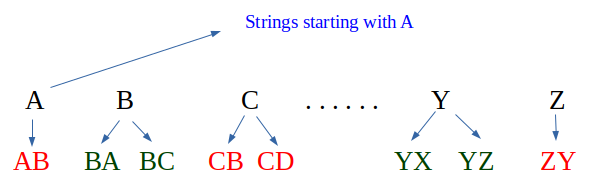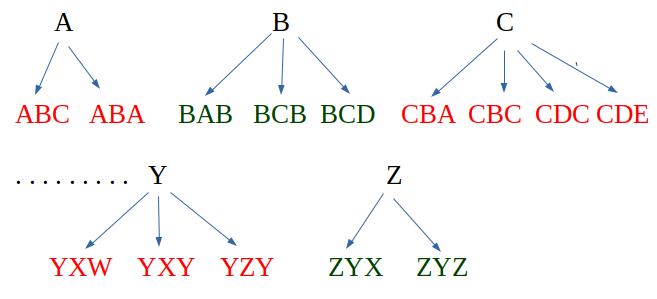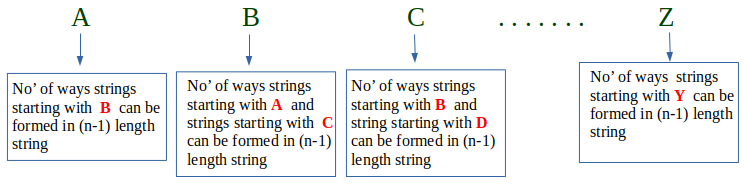Open In App

# Count of strings where adjacent characters are of difference one

Given a number n, count the number of strings of length n such that every string has adjacent characters with a difference between ASCII values as 1.

Examples

```Input :  N = 1
Output : Total strings are 26
Explanation : For N=1, strings
are a, b, c,, ...., x, y, z

Input :  N = 2
Output : Total strings are 50
Explanation : For N = 2, strings
are ab, ba, bc, cb, .., yx, yz, zy```

For strings starting with character ‘A’ and length ‘i’, we consider all strings of length ‘i-1’ and starting with character ‘B’
For strings starting with character ‘G’ and length ‘i’, we consider all strings of length ‘i-1’ and starting with character ‘H’ and all strings of length ‘i-1’ and starting with ‘F’.
We take the base case for n = 1, and set result for all 26 characters as 1. This simply means when 1 character string is consider all alphabets from a-z are taken only once.
For N = 2For N = 3Conclusion : For N = n```countAdjacent(n)
dp[i][j] finally stores count of strings
of length i and starting with
character j.

Initialize dp[n+1] as 0
Initialize dp[j] = 1 where j = 0 to 25
for i = 2 to n
for j = 0 to 25
if (j = 0)
dp[i][j] = dp[i-1][j+1];
else
dp[i][j] = dp[i-1][j-1] + dp[i-1][j+1];
Sum of n-th row from 0 to 25 is the result.```

Implementation:

## C++

 `// CPP Program to count strings with adjacent``// characters.``#include ``using` `namespace` `std;` `int` `countStrs(``int` `n)``{``    ``long` `int` `dp[n + 1];` `    ``// Initializing arr[n+1] to 0``    ``memset``(dp, 0, ``sizeof``(dp));` `    ``// Initializing 1st row all 1 from 0 to 25``    ``for` `(``int` `i = 0; i <= 25; i++)``        ``dp[i] = 1;` `    ``// Begin evaluating from i=2 since 1st row is set``    ``for` `(``int` `i = 2; i <= n; i++) {``        ``for` `(``int` `j = 0; j <= 25; j++)` `            ``// j=0 is 'A' which can make strings``            ``// of length i using strings of length``            ``// i-1 and starting with 'B'``            ``if` `(j == 0)``                ``dp[i][j] = dp[i - 1][j + 1];``            ``else``                ``dp[i][j] = (dp[i - 1][j - 1] +``                            ``dp[i - 1][j + 1]);``    ``}` `    ``// Our result is sum of last row.``    ``long` `int` `sum = 0;``    ``for` `(``int` `i = 0; i <= 25; i++)``        ``sum = (sum + dp[n][i]);``    ``return` `sum;``}` `// Driver's Code``int` `main()``{``    ``int` `n = 3;``    ``cout << ``"Total strings are : "` `<< countStrs(n);``    ``return` `0;``}`

## Java

 `// Java Program to count strings``// with adjacent characters.``import` `java.io.*;``class` `GFG {` `    ``static` `long` `countStrs(``int` `n)``    ``{``        ``long``[][] dp = ``new` `long``[n + ``1``][``27``];` `        ``// Initializing arr[n+1] to 0``        ``for` `(``int` `i = ``0``; i < n + ``1``; i++) {``            ``for` `(``int` `j = ``0``; j < ``27``; j++) {``                ``dp[i][j] = ``0``;``            ``}``        ``}` `        ``// Initializing 1st row all 1 from 0 to 25``        ``for` `(``int` `i = ``0``; i <= ``25``; i++) {``            ``dp[``1``][i] = ``1``;``        ``}` `        ``// Begin evaluating from i=2``        ``// since 1st row is set``        ``for` `(``int` `i = ``2``; i <= n; i++) {` `            ``// j=0 is 'A' which can make strings``            ``for` `(``int` `j = ``0``; j <= ``25``; j++)` `            ``// of length i using strings of length``            ``// i-1 and starting with 'B'``            ``{``                ``if` `(j == ``0``) {``                    ``dp[i][j] = dp[i - ``1``][j + ``1``];``                ``}``                ``else` `{``                    ``dp[i][j] = (dp[i - ``1``][j - ``1``]``                                ``+ dp[i - ``1``][j + ``1``]);``                ``}``            ``}``        ``}` `        ``// Our result is sum of last row.``        ``long` `sum = ``0``;``        ``for` `(``int` `i = ``0``; i <= ``25``; i++) {``            ``sum = (sum + dp[n][i]);``        ``}``        ``return` `sum;``    ``}` `    ``// Driver Code``    ``public` `static` `void` `main(String[] args)``    ``{``        ``int` `n = ``3``;``        ``System.out.println(``"Total strings are : "``                           ``+ countStrs(n));``    ``}``}` `// This code is contributed by 29AjayKumar`

## Python 3

 `# Python3 Program to count strings with``# adjacent characters.``def` `countStrs(n):` `    ``# Initializing arr[n+1] to 0``    ``dp ``=` `[[``0` `for` `j ``in` `range``(``27``)]``             ``for` `i ``in` `range``(n ``+` `1``)]` `    ``# Initializing 1st row all 1 from 0 to 25``    ``for` `i ``in` `range``(``0``, ``26``):``        ``dp[``1``][i] ``=` `1` `    ``# Begin evaluating from i=2 since``    ``# 1st row is set    ``    ``for` `i ``in` `range``(``2``, n ``+` `1``):``        ``for` `j ``in` `range``(``0``, ``26``):` `            ``# j=0 is 'A' which can make strings``            ``# of length i using strings of length``            ``# i-1 and starting with 'B'``            ``if``(j ``=``=` `0``):``                ``dp[i][j] ``=` `dp[i ``-` `1``][j ``+` `1``];``            ``else``:``                ``dp[i][j] ``=` `(dp[i ``-` `1``][j ``-` `1``] ``+``                            ``dp[i ``-` `1``][j ``+` `1``])` `    ``# Our result is sum of last row.        ``    ``sum` `=` `0``    ``for` `i ``in` `range``(``0``, ``26``):``        ``sum` `=` `sum` `+` `dp[n][i]` `    ``return` `sum``    ` `# Driver's Code``if` `__name__ ``=``=` `"__main__"``:``    ``n ``=` `3``    ``print``(``"Total strings are : "``, countStrs(n))``    ` `# This code is contributed by Sairahul Jella`

## C#

 `// C# Program to count strings with ``// adjacent characters.``using` `System;` `class` `GFG``{``    ``static` `long` `countStrs(``int` `n)``    ``{``        ``long``[,] dp = ``new` `long``[n + 1, 27];``    ` `        ``// Initializing arr[n+1] to 0``        ``for``(``int` `i = 0; i < n + 1; i++)``            ``for``(``int` `j = 0; j < 27; j++)``                ``dp[i, j] = 0;``    ` `        ``// Initializing 1st row all 1 from 0 to 25``        ``for` `(``int` `i = 0; i <= 25; i++)``            ``dp[1, i] = 1;``    ` `        ``// Begin evaluating from i=2 since 1st row is set``        ``for` `(``int` `i = 2; i <= n; i++)``        ``{``            ``for` `(``int` `j = 0; j <= 25; j++)``    ` `                ``// j=0 is 'A' which can make strings``                ``// of length i using strings of length``                ``// i-1 and starting with 'B'``                ``if` `(j == 0)``                    ``dp[i, j] = dp[i - 1, j + 1];``                ``else``                    ``dp[i, j] = (dp[i - 1, j - 1] +``                                ``dp[i - 1, j + 1]);``        ``}``    ` `        ``// Our result is sum of last row.``        ``long` `sum = 0;``        ``for` `(``int` `i = 0; i <= 25; i++)``            ``sum = (sum + dp[n, i]);``        ``return` `sum;``    ``}``    ` `    ``// Driver Code``    ``static` `void` `Main()``    ``{``        ``int` `n = 3;``        ``Console.Write(``"Total strings are : "` `+ countStrs(n));``    ``}``}` `// This code is contributed by DrRoot_`

## Javascript

 ``

Output

`Total strings are : 98`

Time Complexity: O(26*n)
Auxiliary Space: O(26*n)

This article is contributed by Shubham Rana. If you like GeeksforGeeks and would like to contribute, you can also write an article using write.geeksforgeeks.org or mail your article to review-team@geeksforgeeks.org. See your article appearing on the GeeksforGeeks main page and help other Geeks.